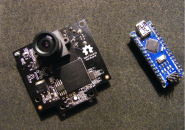## 智能机器人手臂–第2部分：编程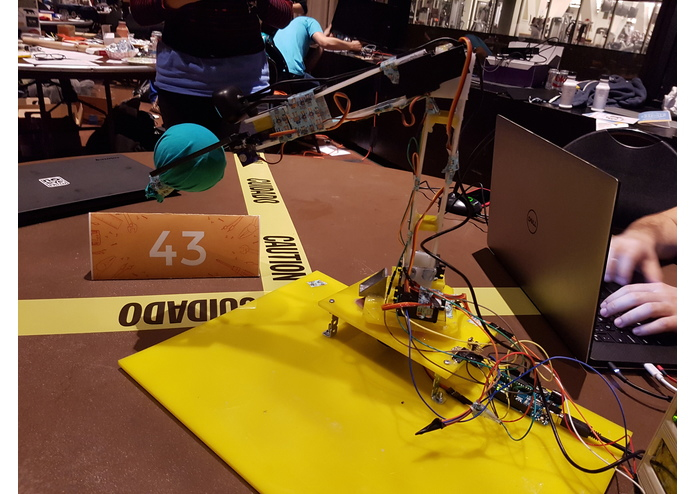• Arduino Uno
• 麦克风
• 网络摄像头
• 伺服电机

## 第1步：制作App

1. 下载EmguCV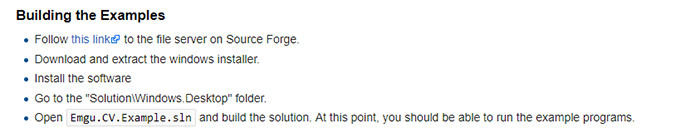2. 添加文件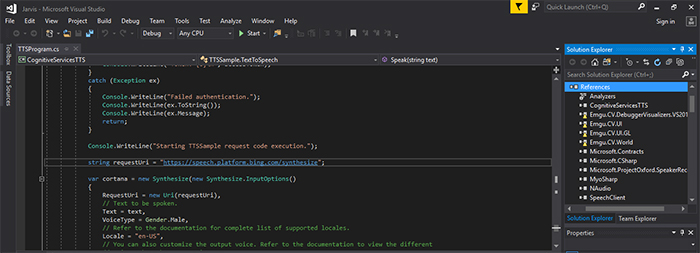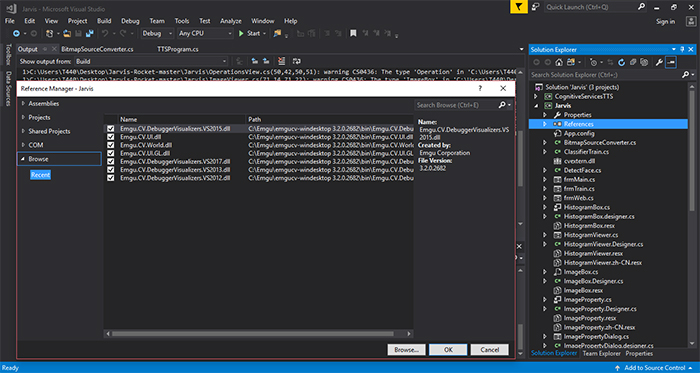3. 示例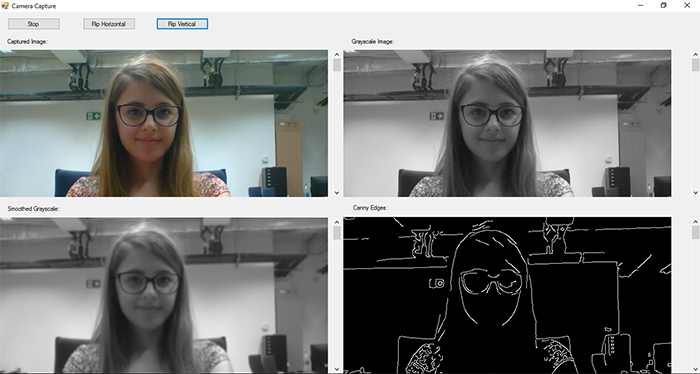4. Arduino程序

Arduino代码具有4个命令：up/down负责控制伺服电机；reset是指在出现问题时重置机器人手臂位置；某个部件没有动作时则使用do nothing。程序具有四个自由度:

• circle – 手臂可以旋转;
• base – 调整网络摄像头的倾斜度;
• elbow– 旨在获取手臂的最佳位置;
• wrist – 调整抓握力.

if(cmd == ‘A’) state = 1; control(1, posCircle);
if(cmd == ‘B’) state = 2; control(2, posBase);
if(cmd == ‘C’) state = 3; control(3, posElbow);
if(cmd == ‘D’) state = 4; control(4, posGH);

Case 1中，Circle（旋转）运动的命令为left/right（左/右）。

Case 1:

``````      switch (cmd)
{
case '0':
Left();
Break;
case '1':
//do nothing
break;
case '2':
Right();
break;
case '3':
posCircle = 0;
break;
}
state = 0;
break;
``````

Case 2和Case 3中，我们考虑基座和肘部的伺服电机，这意味着他们可以上/下（up/down）引导手臂；遇到错误时，机器人手臂会把自己设置为posBase = 50；posElbow = 50;

Case 2:

``````      switch (cmd)
{
case '0':
downBase();
break;

case '1':
//do nothing
break;

case '2':
upBase();
break;

case '3':
posBase = 50;
break;
}
state = 0;
break;
``````

Case 3:

``````      switch (cmd)
{
case '0':
downElbow();
break;

case '1':
//do nothing
break;

case '2':
upElbow();
break;

case '3':
posElbow = 50;
break;

case '4':
posElbow = 10;
break;
}
state = 0;
break;
``````

Case 4中，我们需要控制爪钳，这个非常简单——将其初始位置设置为posGH = 50;

``````// States for the RoboticArm:
//0 Down on the left
//1 Pause
//2 Top on the right
//3 Reset

#include <Servo.h>

Servo Circle; //1
Servo Base; //2
Servo Elbow;  //3
Servo Wrist; //4

int posCircle = 0;
int posBase = 50;
int posElbow = 50;
int posGH = 50;

int state = 0;

void setup() {
Serial.begin(9600);
Elbow.attach(7);
Base.attach(8);
Circle.attach(9);
Wrist.attach(10);
delay(1000);
control(1, posCircle);
control(2, posBase);
control(3, posElbow);
control(4, posGH);
}

void loop()
{

if(Serial.available() > 0)
{

switch (state) {
case 0:
if(cmd == 'A') state = 1;
if(cmd == 'B') state = 2;
if(cmd == 'C') state = 3;
if(cmd == 'D') state = 4;

break;

case 1:

switch (cmd)
{
case '0':
Left();
break;

case '1':
//do nothing
break;

case '2':
Right();
break;

case '3':
posCircle = 0;
break;
}
state = 0;
break;

case 2:

switch (cmd)
{
case '0':
downBase();
break;

case '1':
//do nothing
break;

case '2':
upBase();
break;

case '3':
posBase = 50;
break;
}
state = 0;
break;

case 3:

switch (cmd)
{
case '0':
downElbow();
break;

case '1':
//do nothing
break;

case '2':
upElbow();
break;

case '3':
posElbow = 50;
break;

case '4':
posElbow = 10;
break;
}
state = 0;
break;

case 4:

switch (cmd)
{
case '0':
break;

case '1':
break;

case '2':
break;

case '3':
posGH = 50;
break;
}
state = 0;
break;

}

}

}

void control(int motor, int angle)
{
switch (motor) {
case 1:
if(angle >= 0) if(angle <= 140) Circle.write(angle + 10);
break;

case 2:
if(angle >= 0) if(angle <= 60) Base.write(140 - angle);
break;

case 3:
if(angle >= 0) if(angle <= 70) Elbow.write(80 - angle);
break;

case 4:
if(angle >= 0) if(angle <= 65) Wrist.write(75 - angle);
break;
}
}

void Left()
{
if(posCircle <= 136)
{
posCircle = posCircle + 4;
}
else posCircle = 140;
control(1, posCircle);
}

void Right()
{
if(posCircle >= 4)
{
posCircle = posCircle - 4;
}
else posCircle = 0;
control(1, posCircle);
}

///////////////////////////////////
void downElbow()
{
if(posElbow >= 4)
{
posElbow = posElbow - 4;
}
else posElbow = 0;
control(3, posElbow);
}

void upElbow()
{
if(posElbow <= 66)
{
posElbow = posElbow + 4;
}
else posElbow = 70;
control(3, posElbow);
}
//////////////////////////////////////
void downBase()
{
if(posBase >= 4)
{
posBase = posBase - 4;
}
else posBase = 0;
control(2, posBase);
}

void upBase()
{
if(posBase <= 56)
{
posBase = posBase + 4;
}
else posBase = 60;
control(2, posBase);
}
``````

## 第2步：如何制作演示App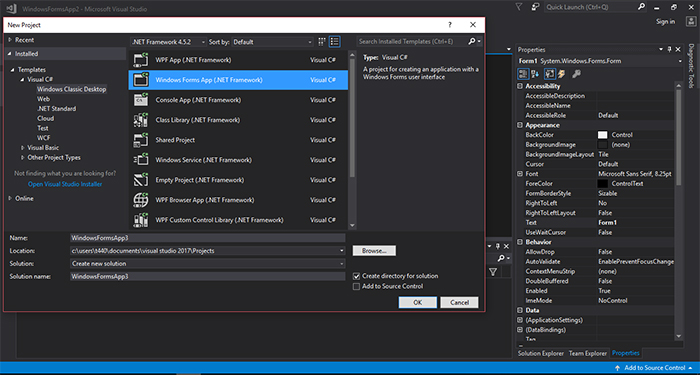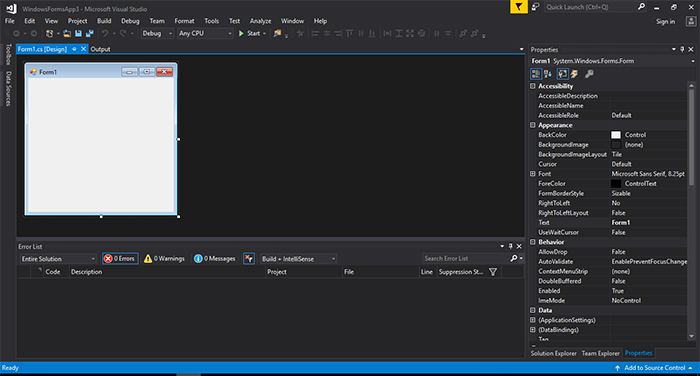``````namespace WindowsFormsApp3
{
public partial class Form1 : Form
{
public Form1()
{
InitializeComponent();
}

private void button1_Click(object sender, EventArgs e)
{
}
}
}
``````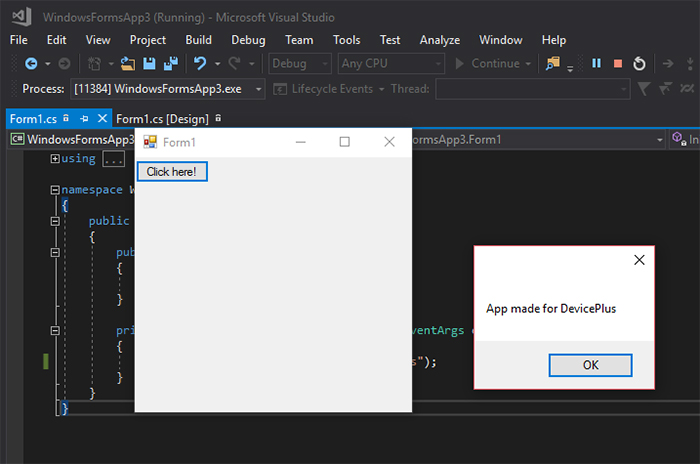## 第3步：App属性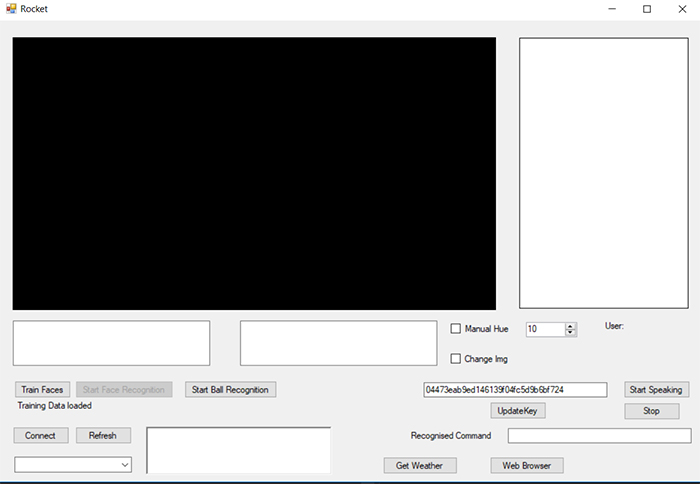## 第4步：人脸识别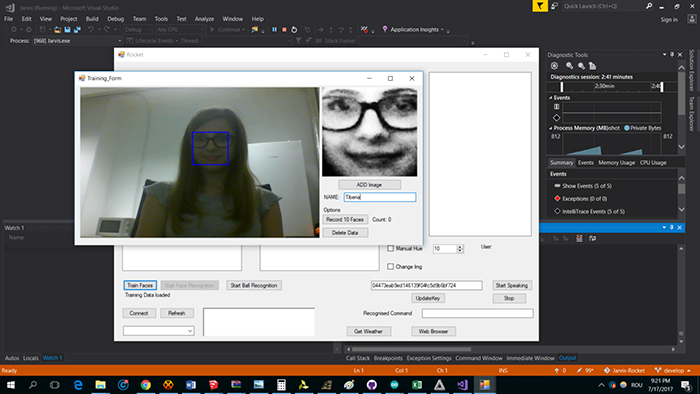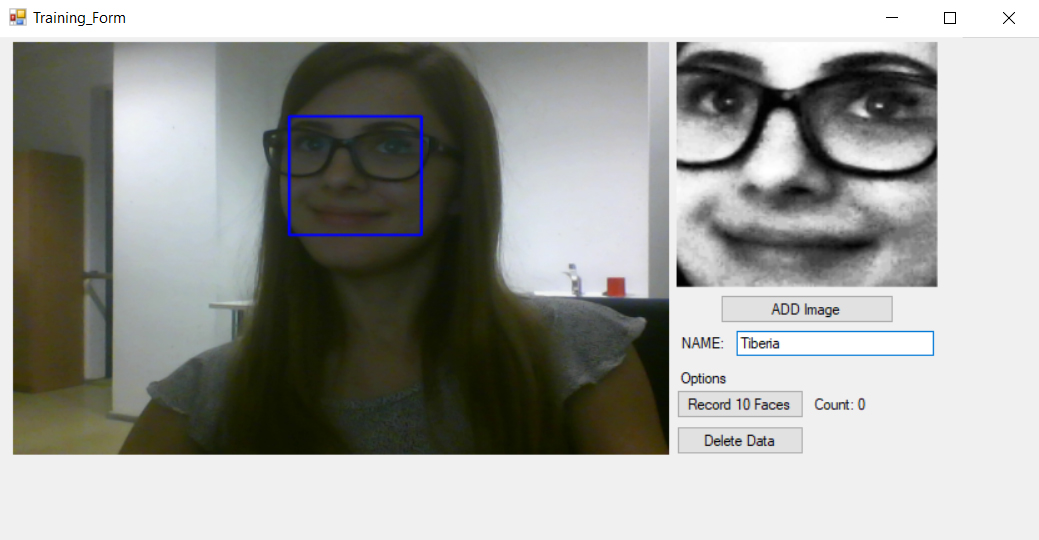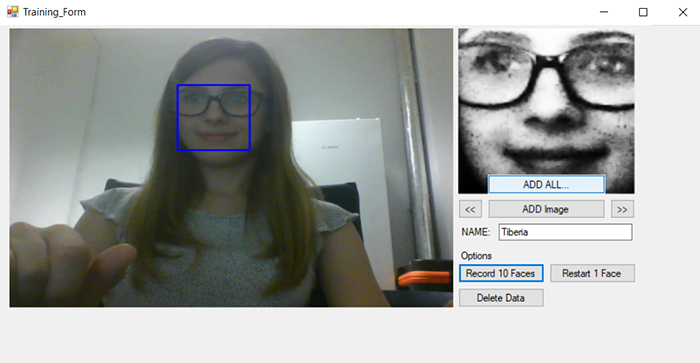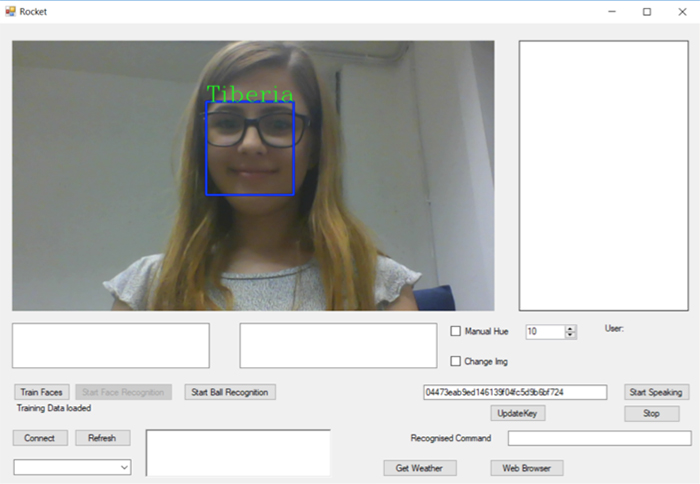## 第5步：天气API

WebClient client = new WebClient();

## 第6步：语音合成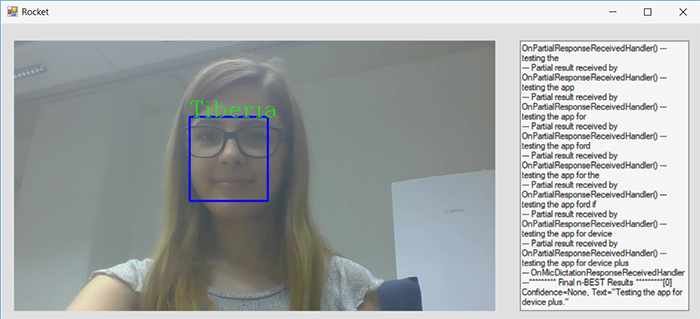##### Tiberia Todeila

Tiberia目前是布加勒斯特理工大学电气工程学院的大四学生。她非常热衷于智能家居设备的设计和开发，旨在让我们的日常生活更加轻松。

### 相关文章

1.2. #### 用Arduino Uno制作一个智能的自动宠物喂食器（续）

3.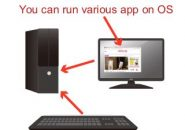#### Arduino基础：Arduino的前期准备

4.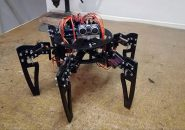#### Arduino六足机器人第二部分：编程

5.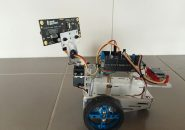#### 利用Parallax激光传感器制作Arduino激光机器人—第1部分

6.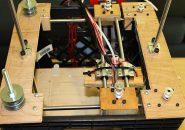7. #### TFT液晶面板与三轴加速度计的配合使用–第2部分

8.